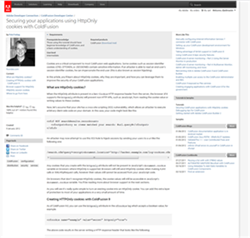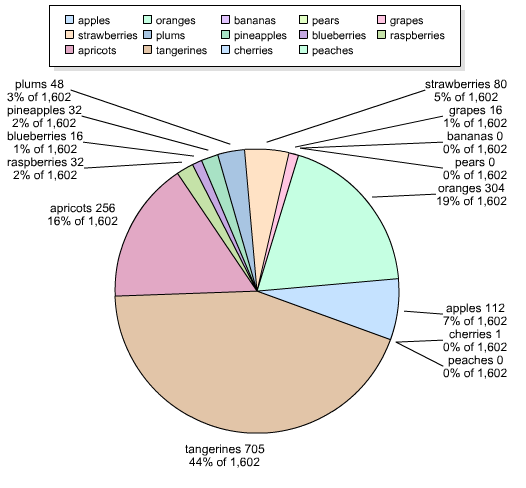I am using cf9 report builder to generate a PDF of a pie chart with many slices (i.e. 14) . When I select 'data label: pattern', for my pie chart, the labels overlap. I have tried changing the size and style (e.g. sliced instead of solid) to no avail. The labels on the pie chart still overlap. I have increased the width and height as much as possible, but the chart is displayed with a page header and footer, so I am unable to use the entire width/height of the page. Is there at least a way to modify the legend so that I might add the [data label: pattern] (e.g. itemName n x% of y) information there and remove the labels from the pie chart altogether?

Unfortunately the last time I used ColdFusion's Report Builder I was a lot less gray and I think HomeSite+ was the best ColdFusion editor. Luckily though I was able to reproduce her issue with a vanilla cfchart and move from there. She sent me fruit data (why not beer???) that I turned into a query like so.

`<cfset q = queryNew("fruit,total","cf_sql_varchar,cf_sql_integer")> <cfset queryAddRow(q)> <cfset querySetCell(q, "fruit","apples")> <cfset querySetCell(q, "total",112)> <cfset queryAddRow(q)> <cfset querySetCell(q, "fruit","oranges")> <cfset querySetCell(q, "total",304)> <cfset queryAddRow(q)> <cfset querySetCell(q, "fruit","bananas")> <cfset querySetCell(q, "total",0)> <cfset queryAddRow(q)> <cfset querySetCell(q, "fruit","pears")> <cfset querySetCell(q, "total",0)> <cfset queryAddRow(q)> <cfset querySetCell(q, "fruit","grapes")> <cfset querySetCell(q, "total",16)> <cfset queryAddRow(q)> <cfset querySetCell(q, "fruit","strawberries")> <cfset querySetCell(q, "total",80)> <cfset queryAddRow(q)> <cfset querySetCell(q, "fruit","plums")> <cfset querySetCell(q, "total",48)> <cfset queryAddRow(q)> <cfset querySetCell(q, "fruit","pineapples")> <cfset querySetCell(q, "total",32)> <cfset queryAddRow(q)> <cfset querySetCell(q, "fruit","blueberries")> <cfset querySetCell(q, "total",16)> <cfset queryAddRow(q)> <cfset querySetCell(q, "fruit","raspberries")> <cfset querySetCell(q, "total",32)> <cfset queryAddRow(q)> <cfset querySetCell(q, "fruit","apricots")> <cfset querySetCell(q, "total",256)> <cfset queryAddRow(q)> <cfset querySetCell(q, "fruit","tangerines")> <cfset querySetCell(q, "total",705)> <cfset queryAddRow(q)> <cfset querySetCell(q, "fruit","cherries")> <cfset querySetCell(q, "total",1)> <cfset queryAddRow(q)> <cfset querySetCell(q, "fruit","peaches")> <cfset querySetCell(q, "total",0)>`

Obviously this would normally be a database query and be a heck of a lot smaller. I then passed this into a pie chart.

`<cfchart chartheight="500" chartwidth="500"> <cfchartseries type="pie" query="q" itemcolumn="fruit" valuecolumn="total" datalabelstyle="pattern" > </cfchart>`

And we can see right away the - um - "suboptimal" rendering:Gross. So I did what I normally do in cases like this and opened up the chart editor. I entered additional data and recreate the issue in their tool. I then played around and discovered that the Data Labels editor has an option called AutoControl. If you mouse over this you see: "Toggles auto control of overlapping labels"

Yeah - no way it's that easy. I turned it on and bam - it worked. So I snagged the XML (and tweaked a few more small things) and got this:

`<?xml version="1.0" encoding="UTF-8"?> <pieChart depth="Double" style="Solid" is3d="false"> <dataLabels style="Pattern" autoControl="true"/> <paint palette="PastelTransluent" paint="Plain" min="44" max="95"/> </pieChart>`

The critical part is autoControl="true". Here is the complete code then to use this XML:

`<cfsavecontent variable="cxml"> <?xml version="1.0" encoding="UTF-8"?> <pieChart depth="Double" style="Solid" is3d="false"> <dataLabels style="Pattern" autoControl="true"/> <paint palette="PastelTransluent" paint="Plain" min="44" max="95"/> </pieChart> </cfsavecontent>`

`<cfchart chartheight="500" chartwidth="500" style="#cxml#"> <cfchartseries type="pie" query="q" itemcolumn="fruit" valuecolumn="total" datalabelstyle="pattern" > </cfchart>`

And the result is:Still "busy" but a heck of a lot more readable. (I'd probably reduce the smallest 5 items into one bucket, but thats for another blog post.) Hope this helps!